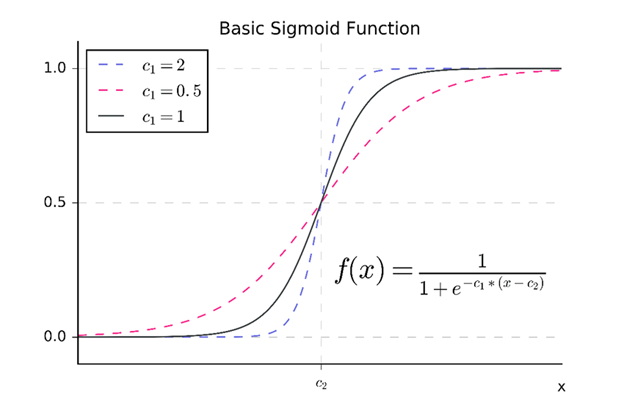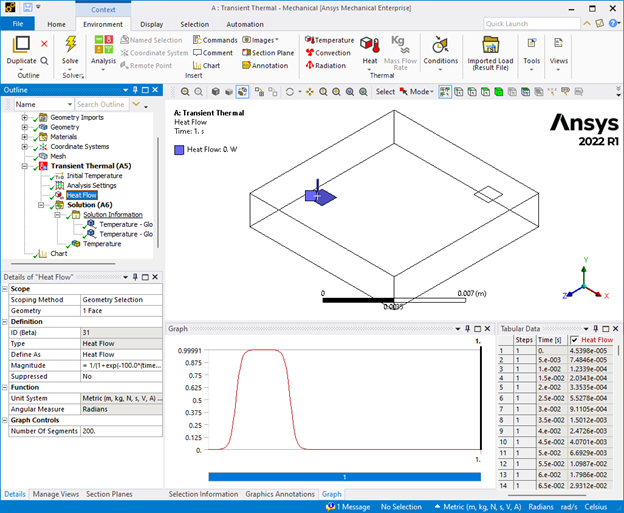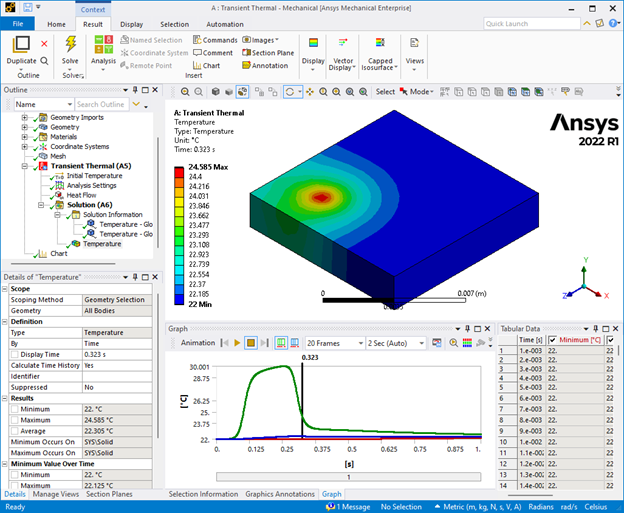Ansys Mechanical allows users to define “Function Loads”. This feature enables time dependent, complex functions and is a good alternative to copy/paste from Excel tables. Ansys Help states the supported functions as: sin/cos/tan/exp/asin/acos/atan/atan2/pow/log/log10/sqrt. I was looking to enter power burst loads in a thermal analysis where I wanted to enter step increase of power loading on some surfaces but as Ansys Help states there was no “step” function. A brief search revealed that I can use the exp function to define a sigmoid function. Sigmoid(logistic) function has a characteristic S shape and can practically be used as a step function. The rate of change and the time instance of the change can be adjusted by means of certain constants used in the function (see figure-1).Figure-1 Sigmoid function (Revkin et.al. 2008)

The solution is not only easy but also gives a means for ramping up in a more smooth way; which may help in achieving convergence. For a pulsatile heat input on a surface one can apply the sigmoid function multiple time just by concatenating as many as needed. In below example the ramp up time (see figure-2).

1/(1+exp(-100.0*(time-0.1)))-(1/(1+exp(-100.0*(time-0.3))))

The advantage of using functions over tabular data is when the solver bisects to smaller time steps and/or for any reason the time step size is changed. With functional loads the fidelity of the load is captured at all time step sizes and instances. This way the temperature profile can be captured accurately with smooth responses (see figure-3).Figure-3 Transient thermal temperature distribution results